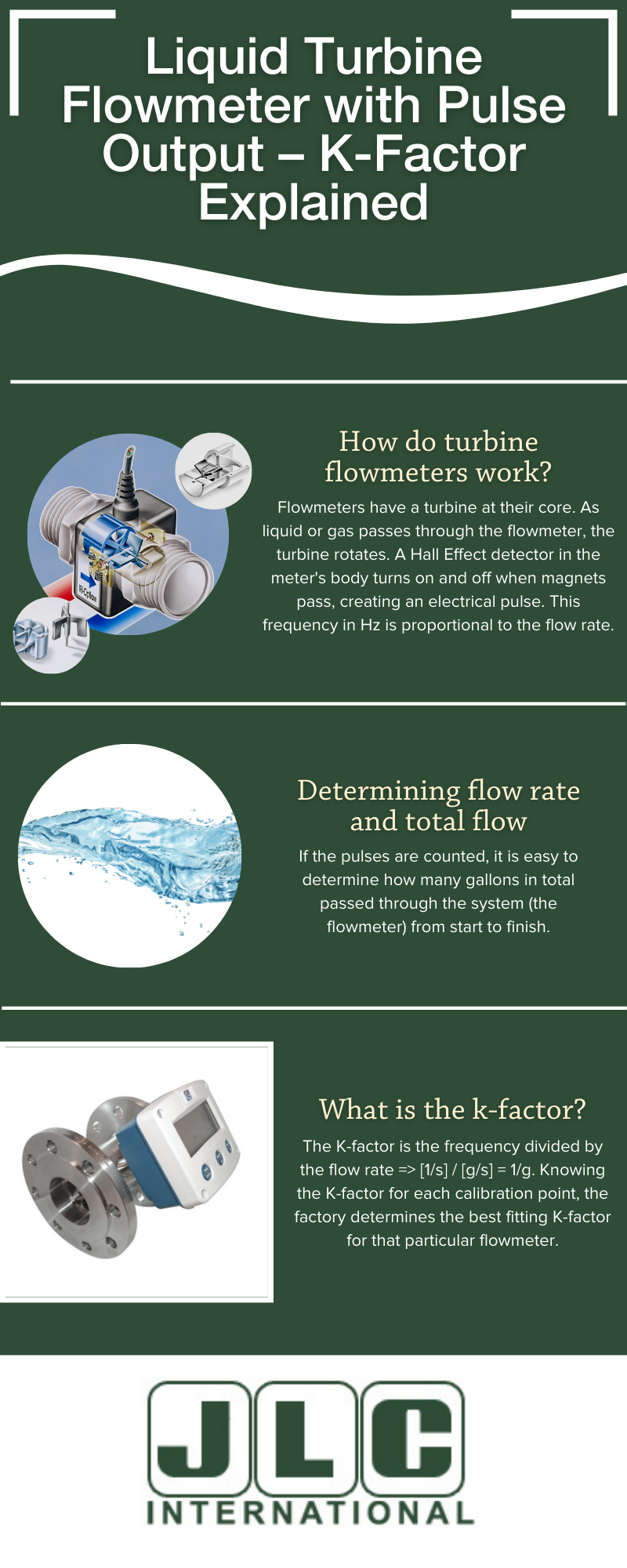# Liquid Turbine Flowmeter with Pulse Output – K-Factor Explained

JLC International has been known for many years for selling the well-known liquid turbine flowmeters of the series 800, 900, and 1000. Our liquid turbine flowmeters are great for all kinds of applications, from dosing chemicals in agriculture spraying machines to measuring the few ounces of a liquor shot in a casino.## How do liquid turbine flowmeters work?The flowmeter has a precision turbine at its core. The turbine rotates almost frictionlessly in its robust sapphire bearings. The embedded ceramic magnets in the turbine blades are encapsulated and are detected with every turn through the chamber wall by a Hall Effect detector. The Hall Effect detector creates an NPN pulse with a height slightly less than the power supply, which can be between 4.5 to 24 Vdc.

The number of pulses per second is called the frequency. Frequency is measured in 1/sec or Hertz (Hz) and is proportional to the flow. When the flowmeter is calibrated at the factory, the meter produces a certain number of pulses per unit of volume called the K-factor, e.g., 7500 pulses per gallon, depending on the flow range measured on the flowmeter.

## Measuring and Calculating the Flow Rate

It is easy to determine how many gallons in total passed through the flowmeter from start to finish by counting the pulse frequency. If 26,250 pulses are counted, it would mean that 3.5 gallons of liquid have passed through the flow meter. You can calculate the average flow rate in gallons per minute by recording the time it takes for all of the liquid to pass through the flow meter. By monitoring the pulses and timing, you are calculating the flow rate and total flow at any given moment.

## Understanding Flow Meter Calibration with K-Factor

The series 800, 900, and 1000 come with a calibration certificate over six or more points. It provides the flow rate values coming from the calibration rig and the frequency produced by the flowmeter for each calibration point. The devices to create the flow and measure the frequency are very accurate and are called calibration standards. They are checked and calibrated by the designated authorities using higher standards, making all standards traceable to the primary source. In the USA,  the National Institute of Standards and Technology (NIST) is responsible for overseeing flow rate calibration standards. In the UK, The National Physical Laboratory (NPL) fulfills a similar function.

If the flow rate and the frequency are known for each calibration point, the K-factor for that point is determined as well. The K-factor is the frequency divided by the flow rate => [1/s] / [g/s] = 1/g. Knowing the K-factor for each calibration point, the factory determines the best fitting K-factor for that particular flowmeter. Calibration points are determined by averaging all K-factors. The calibration certificate states “the best K-factor,” as well as the conditions when the calibration took place, including temperature, pressure, and liquid used.Every paddle, Pelton wheel, and turbine flowmeter produces a pulse output of some sort, and each flowmeter has its own K-factor — they are all different. Installing a flowmeter means that the frequency device needs to be set up for the frequency range and the K-factor of that particular flowmeter.

## K-Factor Calibration Is Essential For Accuracy

Only after the K-factor is known can the frequency counter indicate the flow rate and the total flow, and only then is the system accurate and according to specifications. If a flowmeter has to be replaced sometime in the future, the frequency counter must be reprogrammed with the new K-factor. If that has not been done, the new flowmeter will run against the wrong K-factor, and the indication of the flow rate and total flow will be several percentages off, which can easily be 5 – 10 %.

If many flowmeters have to be installed on, for instance, a spraying machine, each set of flowmeter and frequency counter is set for a different K-factor. Coming from the same batch (shipment) of flowmeters, most likely, the K-factors are close, but not the same. It is laborious and cumbersome to program each frequency counter with different settings, but it is necessary to achieve certain accuracy.

However, for these multiple turbine flowmeter applications, there is now a very clever solution. We have seen that the frequency, flow rate, and K-factor are proportional. Therefore, if an additional onboard electronic package can scale the frequency output proportional to the flow, the K-factor is determined. And if the K-factor can be set to be the same value for each flowmeter of a batch of a very large number of flowmeters, each installation is identical.

The so-called Pre-Scaler board fits inside the flowmeter housing of the series 800, 900, and 1000 and receives the pulses directly from the Hall Effect detector. As described above, as the flowmeter is calibrated at the factory, the K-factor is determined. The Pre-Scaler software demands the input of the actual K-factor and the (fixed) required K-factor; after that, the software does the rest.